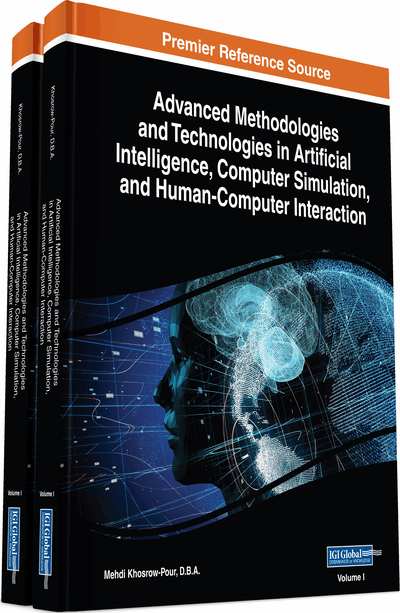# Incremental Approach to Classification Learning

DOI: 10.4018/978-1-5225-7368-5.ch010
OnDemand:
(Individual Chapters)
Available
\$37.50
No Current Special Offers

## Abstract

An approach to incremental classification learning is proposed. Classification learning is based on approximation of a given partitioning of objects into disjointed blocks in multivalued space of attributes. Good approximation is defined in the form of good maximally redundant classification test or good formal concept. A concept of classification context is introduced. Four situations of incremental modification of classification context are considered: adding and deleting objects and adding and deleting values of attributes. Algorithms of changing good concepts in these incremental situations are given and proven.
Chapter Preview
Top

## Introduction

By classification we mean partition of a given object’s set into disjoint blocks or classes. We assume that objects are described by a set U of symbolic or numeric attributes and each object can have one and only one value of each attribute. Then each attribute generates, by its values, partition of a given set of objects into mutually disjoint classes the number of which is equal to the number of values of this attribute. To give a target classification of objects, we use an additional attribute KL not belonging to U. In Table 1, we have two classes: KL+ (positive objects) and KL− (negative objects).

By classification learning we mean approximation of given object classification in terms of attributes names or values of attributes (Naidenova, 2012). This approximation is reduced to extracting logical rules in the form of functional or implicative dependencies from observable datasets. These dependencies allow to distinguish between classes of given classification. For our example in Table 1, we have some rules based on implicative (ID) and functional dependencies (FD): Color_of_Hairs, Color_of_Eyes → KL (FD); if Blond, Blue, then KL = “+”; if Hazel, then KL = “−”; if Brown, Blue, then KL = “−” (IDs).

The task of classification learning based on inferring implicative rules is equivalent to the task of concept formation (Banerji, 1969, Ganter & Wille, 1999). The goal of this task is to describe/classify new objects according to description/classification of existing objects. Inferring good diagnostic (classification) tests (GDTs) is the formation of the best descriptions of a given object class KL+ against the objects not belonging to this class (KL−).

Let M = (∪dom(attr), attr ∈ U), where dom(attr) is the set of all values of attr. Let X ⊆ M and G be the set of indices of objects considered (objects for short), G = G+ ∪ G−, where G+ and G− the sets of positive and negative objects, respectively. Denote by d(g) the description of object g ∈ G. Let P(X) = {g | g ∈ G, X ⊆ d(g)). We call P(X) the interpretation of X in the power set 2G. If P(X) contains only positive objects and the number of these objects more than 2, then we call X a description of some positive objects and (P(X), X) a test for positive objects. Let us define a good test or good description of objects.

Table 1.
Example of classification
 Index of Example Height Color of Hair Color of Eyes KL 1 Low Blond Blue + 2 Low Brown Blue − 3 Tall Brown Hazel − 4 Tall Blond Hazel − 5 Tall Brown Blue − 6 Low Red Blue − 7 Tall Red Blue + 8 Tall Blond Blue +

## Complete Chapter List

Search this Book:
Reset